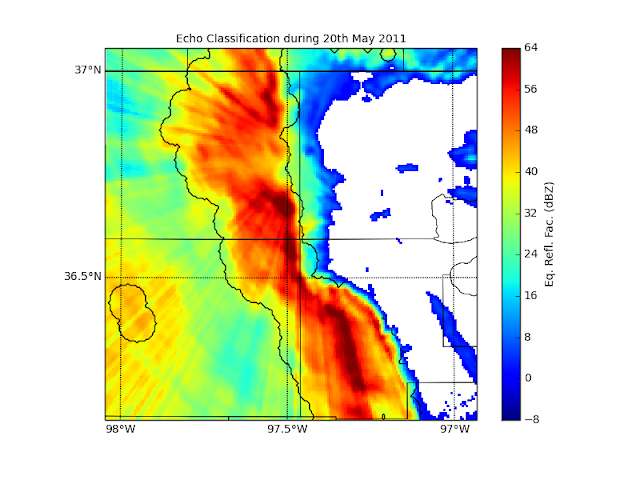## Friday, August 2, 2013

### Introduction

I have been using the Steiner et. al (1995) algorithm for echo classification for some time now. It has its drawbacks, like any algorithm. For example, there are many free parameters that are region dependent, like the automatic convection parameter and the peakedness parameter ($$\Delta Z$$). These parameters, as one might suspect, would have entirely different values for convection near Darwin, Australia than convection near Oklahoma City, Oklahoma.

This algorithm was designed and tuned for radar data collected in Darwin, Australia during February 1988. Therefore, if I want to apply this algorithm to data collected at ARM's Southern Great Plains (SGP) site near Lamont, Oklahoma, I need to first check the results using the default parameters suggested by Steiner et al. (1995), and then tune these parameters accordingly if need be.

It should be noted that this algorithm is applied to gridded reflectivity data at a height chosen to be below the melting layer. In the case of Steiner et al. (1995), they used grids 240 x 240 km in $$x$$ and $$y$$, with 2 km resolution in each dimension. Here I will be using 100 x 100 km grids in $$x$$ and $$y$$, with 500 m resolution in each dimension.

### Methodology

The algorithm is based on three criteria: intensity, peakedness, and surrounding area. The intensity criterion is a simple threshold, where any grid point with a reflectivity of at least 40 dBZ is automatically convective. The peakedness criterion ($$\Delta Z$$) checks the difference between the grid point and the average reflectivity taken over the surrounding background ($$Z_{bg}$$). If this difference is greater than a certain value, then the grid point is labelled convective. Finally, any grid points within an intensity-dependent radius ($$R_{sa}$$) from those already labelled as convective by either the intensity or the peakedness criteria are also labelled convective.

The default parameters defined in Steiner et al. (1995) are as follows:

1. Automatically convective: 40 dBZ.
2. Background radius: 11 km.
3.  $$\Delta Z$$ = $$\begin{cases} 10, & \mbox{if } Z_{bg} \lt 0 \newline 10 - \frac{Z_{bg}^2}{180}, & \mbox{if } 0 \le Z_{bg} \lt 42.43 \newline 0, & \mbox{if } Z_{bg} \ge 42.43 \end{cases}$$
4. There are three different relations for $$R_{sa}$$, the small, medium, and large relations, but each has 1 km and 5 km as the smallest and largest radii, respectively.

### Results

So let's first check how the algorithm, with its default parameters, classifies a squall line event during May 20th, 2011. Near 1033 UTC the squall line, with an axis slightly offset from a north-south direction, was located approximately in the middle of the analysis domain, as it moved through in a west-east direction. There was a leading anvil region to the east and a trailing stratiform region to the west. The results are shown in Fig. 1.Fig. 1. C-SAPR reflectivity and corresponding echo classification at 1033 UTC on May 20th, 2011. The working level (or separation altitude) was a constant 1.5 km. The medium $$R_{sa}$$ relation was used for this classification.

The algorithm does a decent job at classifying the convective squall line feature, however it does miss its leading edge to the east. The trailing stratiform region to the west is another story though. The algorithm is severely affected by brightband contamination here, and a large swath of this region is labelled convective.

One obvious reason for some of the poor classification in the trailing stratiform region is due to the intensity criterion, which was set to 40 dBZ. This value is too weak for convection observed in Oklahoma, which consistently produces reflectivities of 55+ dBZ. Therefore, my first change will be to up this value to 45 dBZ. Another, albeit less obvious reason for the poor classification in the stratiform region is due to the peakedness criterion. Examining the peakedness relation, which varies depending on the intensity of the background reflectivity $$Z_{bg}$$, I see that it does not require a sharp enough gradient between the grid point and $$Z_{bg}$$, and as a result, the brightband contamination, which has a high intensity but at the same time is spatially smooth, gets labelled convective. Therefore, I will use a new relation for $$\Delta Z$$:

$$\Delta Z = \begin{cases} 14, & \mbox{if } Z_{bg} \lt 0 \newline 14 - \frac{Z_{bg}^2}{180}, & \mbox{if } 0 \le Z_{bg} \lt 42.43 \newline 4, & \mbox{if } Z_{bg} \ge 42.43 \end{cases}$$

Basically I have shifted the $$\Delta Z$$ curve up by 4 dBZ, or, in other words, a sharper gradient between the grid point and the background reflectivity is required. The results of these two changes are shown in Fig. 2.Fig. 2. Same as Fig. 1 but tuning the intensity criterion and the peakedness criterion.

#### References

Steiner, M., and R. A. Houze Jr., and S. E. Yuter, 1995: Climatological Characterization of Three-Dimensional Storm Structure from Operational Radar and Rain Gauge Data. J. Appl. Meteor.34, 1978-2007